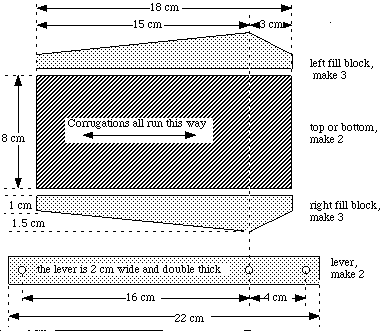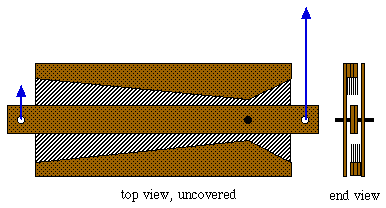# Hands-On-PhysicsMECHANICS

## - Propeller Force -

The force exerted by the propeller is quite small, but it can be measured directly. You should use a variety of measurement tools, like the Thruster, a hanging mass, or a force probe. You could build a force multiplier to amplify the small force produced by the propeller.

Make multiple measurements and calculate the average. Compare your various force measurements and decide which are most accurate. What is your best estimate of the propeller force? What is the range of uncertainty in this estimate?

Force Multiplier
Sometime you want to measure forces which are either too large or too small for your instruments. Forces can be multiplied (or divided) using a lever. A Force Multiplier, to use with the Thruster, can be constructed from cardboard. These are plans for a 4 to 1 multiplier, but these plans could be changed to produce a different ratio.

### Force Multiplier PartsFigure E1
PartsFigure E2
Multiplier with balanced forces

The force multiplier is a double-thick lever inside a sandwich. The top and bottom of the sandwich are held apart by triple-layered spacer blocks on each side of the lever. The lever pivots on a pop-rivet bearing. Forces are applied at the holes in the ends of the lever, perpendicular to the lever.

Previous Page || Up a Level || Index || Next Page